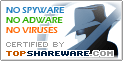# MasterGraph 1.0

SNAPSHOT

Plots graphs of any mathematical functions.

Windows AllPlatform :
USD \$24.95Price :
424 KBFile Size :
ScreenshotScreenshot :Popularity :MasterGraph plots graphs of both simple and exceedingly complex mathematical functions, saves and prints images of graphs, zooms in/out. Screen keyboard lets you to type expressions easy and fast. There are 5 patterns of the most general functions such as "line", "parabola", "hyperbola" etc. Canvas has rich settings of appearance: color, spacing, fonts.

• CalcExp
A Powerful Java Science Calculator.
• ScienCalc
Convenient and powerful scientific calculator
• The Math Professor
The Math Professor 1.5 is the best basic mathematics program
• zsCalculator
Performs calculations, solves expressions, plots graph of functions,...
• AcaStat
Statistical analysis and instructional tool
• smartCalc
orangeCalc 2005 small calculator
• CompactCalc
CompactCalc is enhanced scientific calculator
• Decimal Calculator
DeciCalc is a powerful calculator software